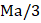# Complete the given number/letter analogy, by choosing the correct answer from the given alternatives. TEKCAR : RACKET : TCEJBO : ? (a) TECOBJ (b) OBJECT (c) CEJBOT (d) REJECT

## Question ID - 100795 :- Complete the given number/letter analogy, by choosing the correct answer from the given alternatives. TEKCAR : RACKET : TCEJBO : ? (a) TECOBJ (b) OBJECT (c) CEJBOT (d) REJECT

3537

OBJECT

Next Question :

A uniform cylinder of massand radiusis placed on a rough horizontal board, which in turn is placed on a smooth surface.The coefficient of friction between the board and the cylinder is. If the board starts accelerating with constant accelerationas shown in the figure, thena) For pure rolling motion of the cylinder, direction of frictional force is forward and its magnitude isb) The maximum value of, so that the cylinder performs pure rolling isc) The acceleration of the center of mass of the cylinder under pure rolling condition for the givenisd) None of these